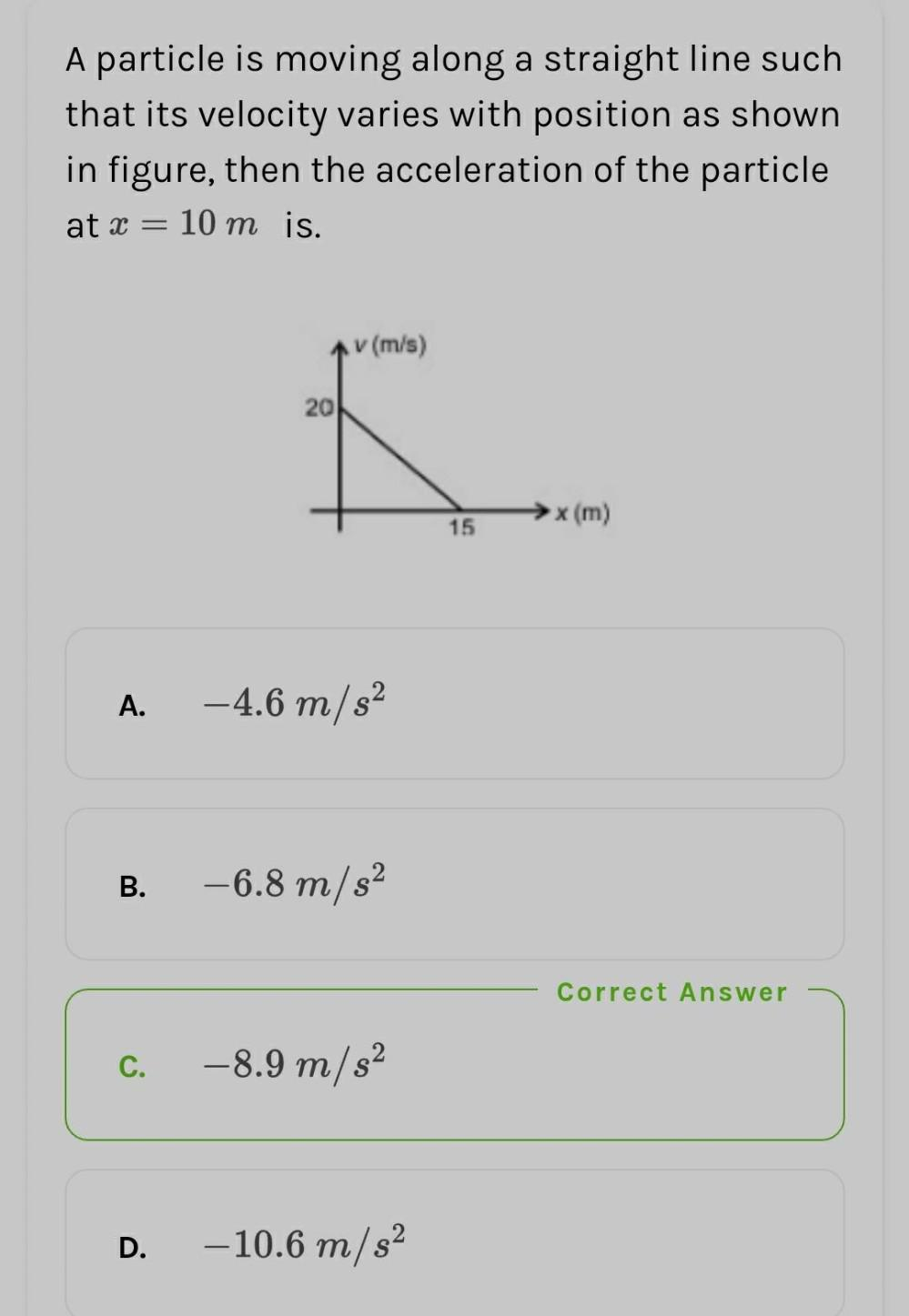Question:

# A particle is moving along a straight line such that its velocity varies with position as shown in figure, then the accelerationA particle is moving along a straight line such that its velocity varies with position as shown in figure, then the acceleration of the particle at x = 10 m is. (m/s) 20 x (m) 15 A. -4.6 m/s2 B. -6.8 m/s2 Correct Answer C. -8.9 m/s2 D. -10.6 m/s2